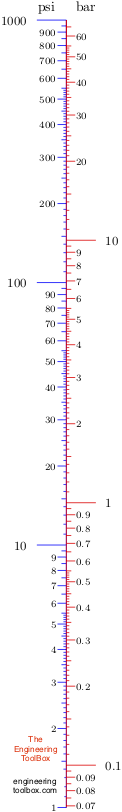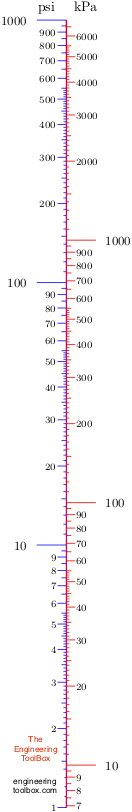Engineering ToolBox - Resources, Tools and Basic Information for Engineering and Design of Technical Applications!

# Pressure Units - Online Converter

## Convert between pressure units like Pa,  bar, atmosphere, pound square feet, psi and more.

### Pressure Converter

The calculator below can be used to convert between some common pressure units

Value (use period as decimal point)

Pa (N/m2)

kPa

bar

atmosphere

mm Hg

mm H2O

m H2O

kgf/m2

kgf/cm2

pound square feet (psf)

pound square inches (psi)

inches Hg

inches H2O

ft H2O

### Pressure Converting Tables

The tables below can be used to convert between some common pressure units:

For full table - rotate the screen!

 Multiply by Convert from Convert to Pa (N/m2) bar atmosphere mm Hg mm H2O m H2O kg/cm2 Pa (N/m2) 1 10-5 9.87 10-6 0.0075 0.1 10-4 1.02 10-5 bar 105 1 0.987 750 1.0197 104 10.197 1.0197 atmosphere 1.01 105 1.013 1 759.9 10332 10.332 1.03 mm Hg 133.3 1.33 10-3 1.32 10-3 1 13.3 0.013 1.36 10-3 mm H2O 10 0.000097 9.87 10-5 0.075 1 0.001 1.02 10-4 m H2O 104 0.097 9.87 10-2 75 1000 1 0.102 kg/cm2 9.8 104 0.98 0.97 735 10000 10 1 pound square feet 47.8 4.78 10-4 4.72 10-4 0.36 4.78 4.78 10-3 4.88 10-4 pound square inches (psi) 6894.76 0.069 0.068 51.7 689.7 0.690 0.07 inches Hg 3377 0.0338 0.033 25.4 337.7 0.337 0.034 inches H2O 248.8 2.49 10-3 2.46 10-3 1.87 25.4 0.0254 0.0025

For full table - rotate the screen!

 Multiply by Convert from Convert to pound square feet pound square inches (psi) inches Hg inches H2O Pa (N/m2) 0.021 1.450326 10-4 2.96 10-4 4.02 10-3 bar 2090 14.50 29.61 402 atmosphere 2117.5 14.69 29.92 407 mm Hg 2.79 0.019 0.039 0.54 mm H2O 0.209 1.45 10-3 2.96 10-3 0.04 m H2O 209 1.45 2.96 40.2 kg/cm2 2049 14.21 29.03 394 pound square feet (psf) 1 0.0069 0.014 0.19 pound square inches (psi) 144 1 2.04 27.7 inches Hg 70.8 0.49 1 13.57 inches H2O 5.2 0.036 0.074 1
• 1 Torr = 1 mm Hg

Note! - pressure is "force per unit area" and commonly used pressure units like kg/cm2 and similar are in principle not correct since kg is a mass unit. Mass must be multiplied with gravity g to be a force (weight).

### Example - Convert from 10 psi to Pa

From the table above - to convert from psi, multiply psi with 6894.76

10 (psi) = 6894.76 10 (Pa) = 68947.6 Pa = 68.95 kPa

### Some other Pressure Units

1 Pa (N/m2) = 0.0000102 Atmosphere (metric)
1 Pa (N/m2) = 0.0000099 Atmosphere (standard)
1 Pa (N/m2) = 0.00001 Bar
1 Pa (N/m2) = 10 Barad
1 Pa (N/m2) = 10 Barye
1 Pa (N/m2) = 0.0007501 Centimeter of mercury (0 C)
1 Pa (N/m2) = 0.0101974 Centimeter of water (4 C)
1 Pa (N/m2) = 10 Dyne/square centimeter
1 Pa (N/m2) = 0.0003346 Foot of water (4 C)
1 Pa (N/m2) = 10-9 Gigapascal
1 Pa (N/m2) = 0.01 Hectopascal
1 Pa (N/m2) = 0.0002953 Inch of mercury (0 C)
1 Pa (N/m2) = 0.0002961 Inch of mercury (15.56 C)
1 Pa (N/m2) = 0.0040186 Inch of water (15.56 C)
1 Pa (N/m2) = 0.0040147 Inch of water (4 C)
1 Pa (N/m2) = 0.0000102 Kilogram force/centimetre2
1 Pa (N/m2) = 0.0010197 Kilogram force/decimetre2
1 Pa (N/m2) = 0.101972 Kilogram force/meter2
1 Pa (N/m2) = 10-7 Kilogram force/millimeter2
1 Pa (N/m2) = 10-3 Kilopascal
1 Pa (N/m2) = 10-7 Kilopound force/square inch
1 Pa (N/m2) = 10-6 Megapascal
1 Pa (N/m2) = 0.000102 Meter of water (4 C)
1 Pa (N/m2) = 10 Microbar (barye, barrie)
1 Pa (N/m2) = 7.50062 Micron of mercury (millitorr)
1 Pa (N/m2) = 0.01 Millibar
1 Pa (N/m2) = 0.0075006 Millimeter of mercury (0 C)
1 Pa (N/m2) = 0.10207 Millimeter of water (15.56 C)
1 Pa (N/m2) = 0.10197 Millimeter of water (4 C)
1 Pa (N/m2) =7.5006 Millitorr
1 Pa (N/m2) = 1 Newton/square meter
1 Pa (N/m2) = 32.1507 Ounce force (avdp)/square inch
1 Pa (N/m2) = 0.0208854 Pound force/square foot
1 Pa (N/m2) = 0.000145 Pound force/square inch
1 Pa (N/m2) = 0.671969 Poundal/square foot
1 Pa (N/m2) = 0.0046665 Poundal/square inch
1 Pa (N/m2) = 0.0000099 Standard atmosphere
1 Pa (N/m2) = 0.0000093 Ton (long)/foot2
1 Pa (N/m2) = 10-7 Ton (long)/inch2
1 Pa (N/m2) = 0.0000104 Ton (short)/foot2
1 Pa (N/m2) = 0.64 10-7 Ton/inch2
1 Pa (N/m2) = 0.0075006 Torr

### psi to bar Nomogram### psi to kPa Nomogram## Related Topics

• ### Basics

The SI-system, unit converters, physical constants, drawing scales and more.
• ### Fluid Mechanics

The study of fluids - liquids and gases. Involving velocity, pressure, density and temperature as functions of space and time.
• ### Pumps

Piping systems and pumps - centrifugal pumps, displacement pumps - cavitation, viscosity, head and pressure, power consumption and more.

## Related Documents

• ### Ammonia - Properties at Gas-Liquid Equilibrium Conditions

Figures and tables showing how the properties of liquid and gaseous ammonia changes along the boiling/condensation curve (temperature and pressure between triple point and critical point conditions). An ammonia phase diagram are included.
• ### Ammonia - Vapour Pressure at Gas-Liquid Equilibrium

Figures and table with ammonia saturation pressure at boiling points, SI and Imperial units.
• ### Barometric Pressure - Pressure in psi, psf and kPa

Convert between barometric pressure inches Hg, psi and psf.
• ### Hazen-Williams Pressure Loss Equation

The Hazen-Williams equation can be used to calculate the pressure drop (psi) or friction loss in pipes or tubes.
• ### Hydrostatic Pressure vs. Depth

Depth and hydrostatic pressure.
• ### Pressure

Introduction to pressure - online pressure units converter.
• ### Pressure Energy

Calculate the potential of pressure energy in a incompressible fluid.
• ### Pressure to Head Unit Converter

Pressure vs. head units - like lb/in2, atm, inches mercury, bars, Pa and more.
• ### Pumps - Head vs. Pressure

Converting head (ft or m) to pressure (psi or bar, kg/cm2) and vice versa.
• ### Steel Pipes Schedule 40 - Pressure Loss

Water flow and pressure loss in schedule 40 steel pipes - Imperial and SI units - gallons per minute, liters per second and cubic meters per hour.
• ### STP - Standard Temperature and Pressure and NTP - Normal Temperature and Pressure

The definition of STP - Standard Temperature and Pressure and NTP - Normal Temperature and Pressure.
• ### Unit Converter with commonly used Units

Common converting units for Acceleration, Area, Density, Energy, Energy per unit mass, Force, Heat flow rate, Heat flux, Heat generation per unit volume and many more.
• ### Unit Factor Method

Convert between units with the unit factor or factor-label method
• ### Water - Properties at Gas-Liquid Equilibrium Conditions

Figures and tables showing how the properties of water changes along the boiling/condensation curve (vapor pressure, density, viscosity, thermal conductivity, specific heat, Prandtl number, thermal diffusivity, entropy and enthalpy).

## Engineering ToolBox - SketchUp Extension - Online 3D modeling!

Add standard and customized parametric components - like flange beams, lumbers, piping, stairs and more - to your Sketchup model with the Engineering ToolBox - SketchUp Extension - enabled for use with older versions of the amazing SketchUp Make and the newer "up to date" SketchUp Pro . Add the Engineering ToolBox extension to your SketchUp Make/Pro from the Extension Warehouse !

We don't collect information from our users. More about

## Citation

• The Engineering ToolBox (2003). Pressure Units - Online Converter. [online] Available at: https://www.engineeringtoolbox.com/pressure-units-converter-d_569.html [Accessed Day Month Year].

Modify the access date according your visit.

9.19.12